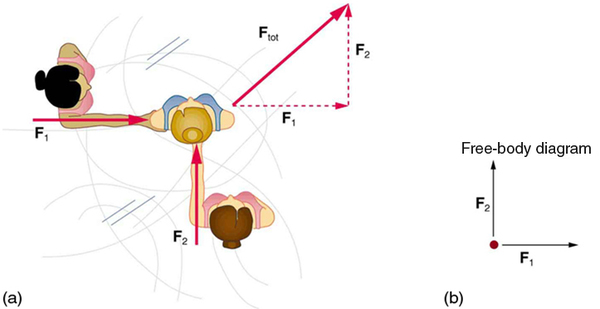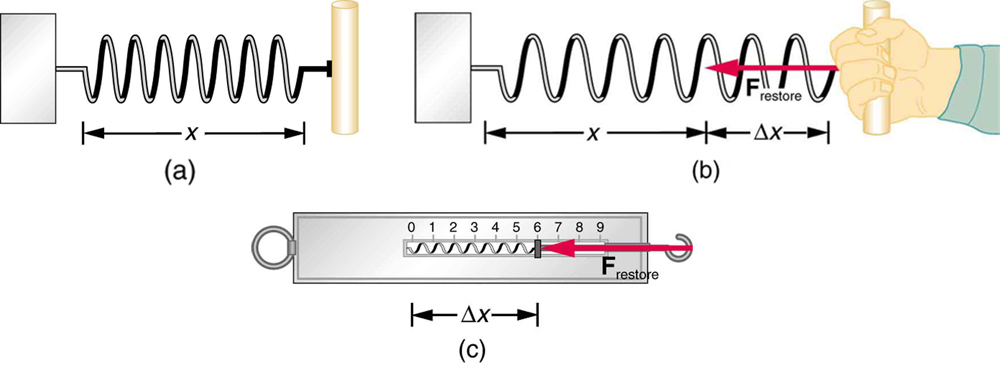# 4.1 Development of force concept

 Page 1 / 5
• Understand the definition of force.

Dynamics is the study of the forces that cause objects and systems to move. To understand this, we need a working definition of force. Our intuitive definition of force    —that is, a push or a pull—is a good place to start. We know that a push or pull has both magnitude and direction (therefore, it is a vector quantity) and can vary considerably in each regard. For example, a cannon exerts a strong force on a cannonball that is launched into the air. In contrast, Earth exerts only a tiny downward pull on a flea. Our everyday experiences also give us a good idea of how multiple forces add. If two people push in different directions on a third person, as illustrated in [link] , we might expect the total force to be in the direction shown. Since force is a vector, it adds just like other vectors, as illustrated in [link] (a) for two ice skaters. Forces, like other vectors, are represented by arrows and can be added using the familiar head-to-tail method or by trigonometric methods. These ideas were developed in Two-Dimensional Kinematics .Part (a) shows an overhead view of two ice skaters pushing on a third. Forces are vectors and add like other vectors, so the total force on the third skater is in the direction shown. In part (b), we see a free-body diagram representing the forces acting on the third skater.

[link] (b) is our first example of a free-body diagram    , which is a technique used to illustrate all the external forces acting on a body. The body is represented by a single isolated point (or free body), and only those forces acting on the body from the outside (external forces) are shown. (These forces are the only ones shown, because only external forces acting on the body affect its motion. We can ignore any internal forces within the body.) Free-body diagrams are very useful in analyzing forces acting on a system and are employed extensively in the study and application of Newton’s laws of motion.

A more quantitative definition of force can be based on some standard force, just as distance is measured in units relative to a standard distance. One possibility is to stretch a spring a certain fixed distance, as illustrated in [link] , and use the force it exerts to pull itself back to its relaxed shape—called a restoring force —as a standard. The magnitude of all other forces can be stated as multiples of this standard unit of force. Many other possibilities exist for standard forces. (One that we will encounter in Magnetism is the magnetic force between two wires carrying electric current.) Some alternative definitions of force will be given later in this chapter.The force exerted by a stretched spring can be used as a standard unit of force. (a) This spring has a length x size 12{x} {} when undistorted. (b) When stretched a distance Δ x size 12{Dx} {} , the spring exerts a restoring force, F restore size 12{F rSub { size 8{"restore"} } } {} , which is reproducible. (c) A spring scale is one device that uses a spring to measure force. The force F restore size 12{F rSub { size 8{"restore"} } } {} is exerted on whatever is attached to the hook. Here F restore size 12{F rSub { size 8{"restore"} } } {} has a magnitude of 6 units in the force standard being employed.

## Take-home experiment: force standards

To investigate force standards and cause and effect, get two identical rubber bands. Hang one rubber band vertically on a hook. Find a small household item that could be attached to the rubber band using a paper clip, and use this item as a weight to investigate the stretch of the rubber band. Measure the amount of stretch produced in the rubber band with one, two, and four of these (identical) items suspended from the rubber band. What is the relationship between the number of items and the amount of stretch? How large a stretch would you expect for the same number of items suspended from two rubber bands? What happens to the amount of stretch of the rubber band (with the weights attached) if the weights are also pushed to the side with a pencil?

## Section summary

• Dynamics is the study of how forces affect the motion of objects.
• Force is a push or pull that can be defined in terms of various standards, and it is a vector having both magnitude and direction.
• External forces are any outside forces that act on a body. A free-body diagram    is a drawing of all external forces acting on a body.

## Conceptual questions

Propose a force standard different from the example of a stretched spring discussed in the text. Your standard must be capable of producing the same force repeatedly.

What properties do forces have that allow us to classify them as vectors?

#### Questions & Answers

What does mean ohms law imply
what is matter
Anything that occupies space
Kevin
Any thing that has weight and occupies space
Victoria
Anything which we can feel by any of our 5 sense organs
Suraj
Right
Roben
thanks
Suraj
what is a sulphate
Alo
Alo
the time rate of increase in velocity is called
acceleration
Emma
What is uniform velocity
Victoria
Greetings,users of that wonderful app.
how to solve pressure?
how do we calculate weight and eara eg an elefant that weight 2000kg has four fits or legs search of surface eara is 0.1m2(1metre square) incontact with the ground=10m2(g =10m2)
Cruz
P=F/A
Mira
can someone derive the formula a little bit deeper?
Bern
what is coplanar force?
what is accuracy and precision
How does a current follow?
follow?
akif
which one dc or ac current.
akif
how does a current following?
Vineeta
?
akif
AC current
Vineeta
AC current follows due to changing electric field and magnetic field.
akif
you guys are just saying follow is flow not follow please
Abubakar
ok bro thanks
akif
flows
Abubakar
but i wanted to understand him/her in his own language
akif
but I think the statement is written in English not any other language
Abubakar
my mean that in which form he/she written this,will understand better in this form, i write.
akif
ok
Abubakar
ok thanks bro. my mistake
Vineeta
u are welcome
Abubakar
what is a semiconductor
substances having lower forbidden gap between valence band and conduction band
akif
what is a conductor?
Vineeta
replace lower by higher only
akif
convert 56°c to kelvin
Abubakar
How does a current follow?
Vineeta
A semiconductor is any material whose conduction lies between that of a conductor and an insulator.
AKOWUAH
what is Atom? what is molecules? what is ions?
What is a molecule
Is a unit of a compound that has two or more atoms either of the same or different atoms
Justice
A molecule is the smallest indivisible unit of a compound, Just like the atom is the smallest indivisible unit of an element.
Rachel
what is a molecule?
Vineeta
what is a vector
A quantity that has both a magnitude AND a direction. E.g velocity, acceleration, force are all vector quantities. Hope this helps :)
deage
what is the difference between velocity and relative velocity?
Mackson
Velocity is the rate of change of displacement with time. Relative velocity on the other hand is the velocity observed by an observer with respect to a reference point.
Chuks
what do u understand by Ultraviolet catastrophe?
Rufai
A certain freely falling object, released from rest, requires 1.5seconds to travel the last 30metres before it hits the ground. (a) Find the velocity of the object when it is 30metres above the ground.
Mackson
A vector is a quantity that has both magnitude and direction
Rufus
the velocity Is 20m/s-2
Rufus
derivation of electric potential
V = Er = (kq/r^2)×r V = kq/r Where V: electric potential.
Chuks
what is the difference between simple motion and simple harmonic motion ?
syed
hi
Peace
hi
Rufus
hi
Chip
simple harmonic motion is a motion of tro and fro of simple pendulum and the likes while simple motion is a linear motion on a straight line.
Muinat
a body acceleration uniform from rest a 6m/s -2 for 8sec and decelerate uniformly to rest in the next 5sec,the magnitude of the deceleration is ?
The wording not very clear kindly
Moses
6
Leo
9.6m/s2
Jolly
the magnitude of deceleration =-9.8ms-2. first find the final velocity using the known acceleration and time. next use the calculated velocity to find the size of deceleration.
Mackson
wrong
Peace
-3.4m/s-2
Justice
Hi
Abj
Firstly, calculate final velocity of the body and then the deceleration. The final ans is,-9.6ms-2
Muinat
8x6= 48m/-2 use v=u + at 48÷5=9.6
Lawrence
can i define motion like this motion can be define as the continuous change of an object or position
Any object in motion will come to rest after a time duration. Different objects may cover equal distance in different time duration. Therefore, motion is defined as a change in position depending on time.
ChuksByBy Anindyo MukhopadhyayByBy Mariah HauptmanByBy Mistry BhaveshByBy